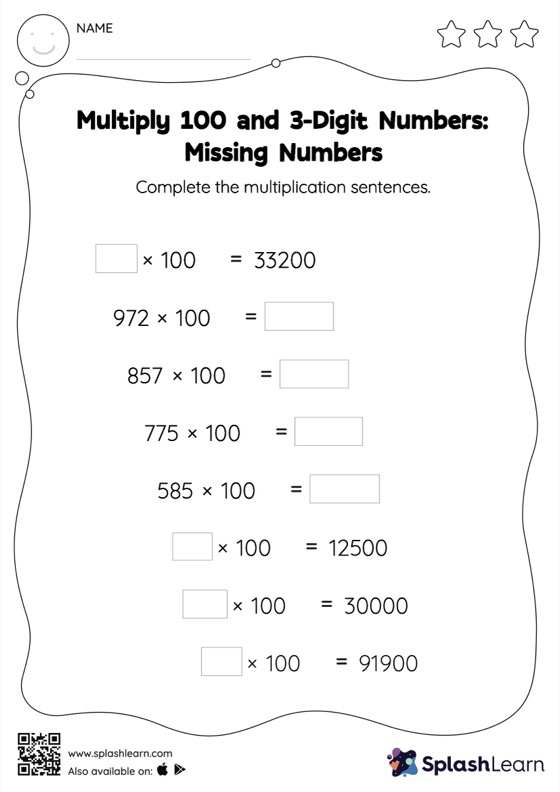# Multiply 100 and 3-Digit Numbers: Missing Numbers Worksheet

Home > Multiply 100 and 3-Digit Numbers: Missing NumbersStudents use the pattern of zeros when they are asked to multiply numbers by 10, 100, or their multiples. They apply the same concept to find the missing number in multiply 100 and 3-digit numbers worksheet. This worksheet is about practicing with the horizontal format in which numbers are written side by side. To develop flexibility with numbers and operations, students need to have enough practice in this format and not just rely on the vertical/column method.